CBSE Class 10 Sample Paper for 2019 Boards

Class 10
Solutions of Sample Papers for Class 10 Boards

Question 27 (OR 1 st question)

A man on the top of a vertical observation tower observes a car moving at a uniform speed coming directly towards it. If it takes 12 minutes for the angle of depression to change from 30° to 45°,  how long will the car take to reach the observation tower from this point?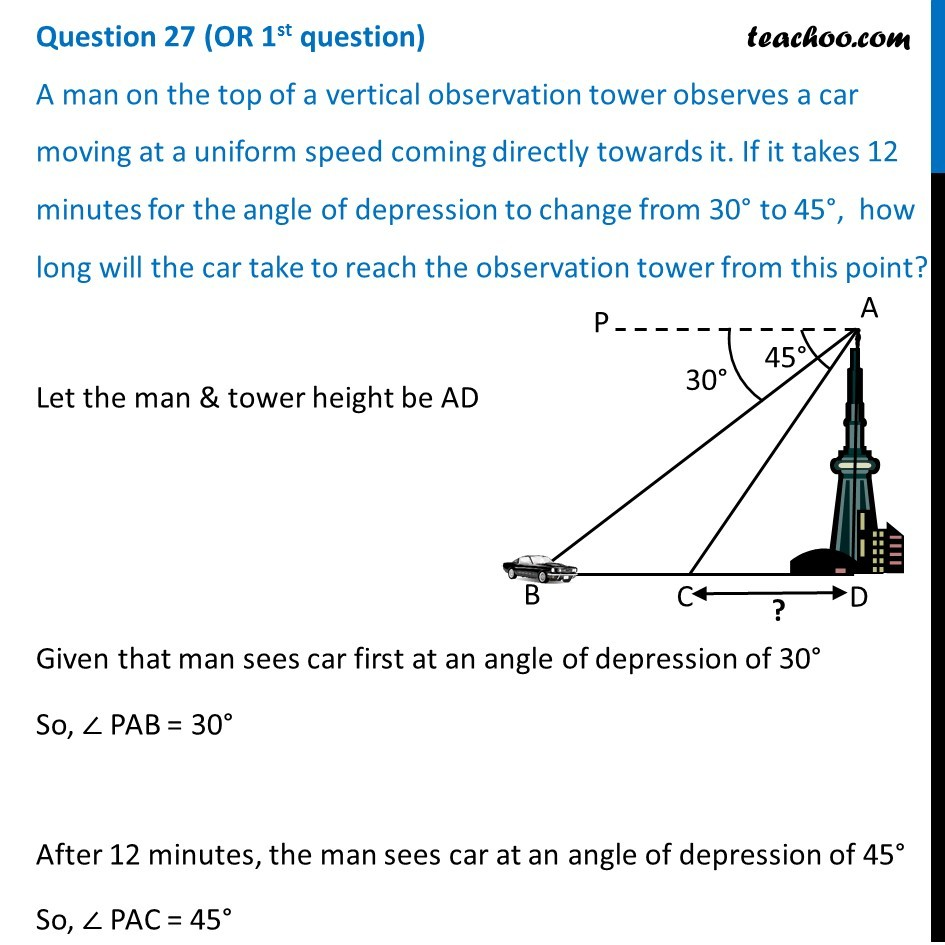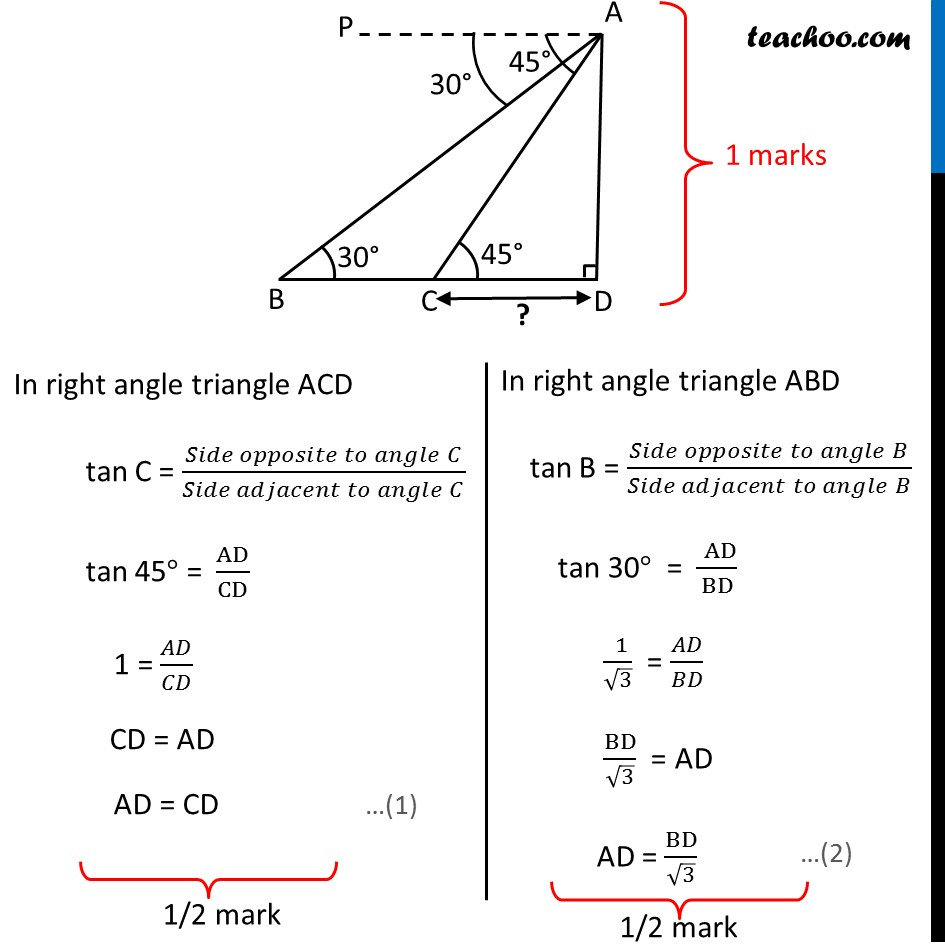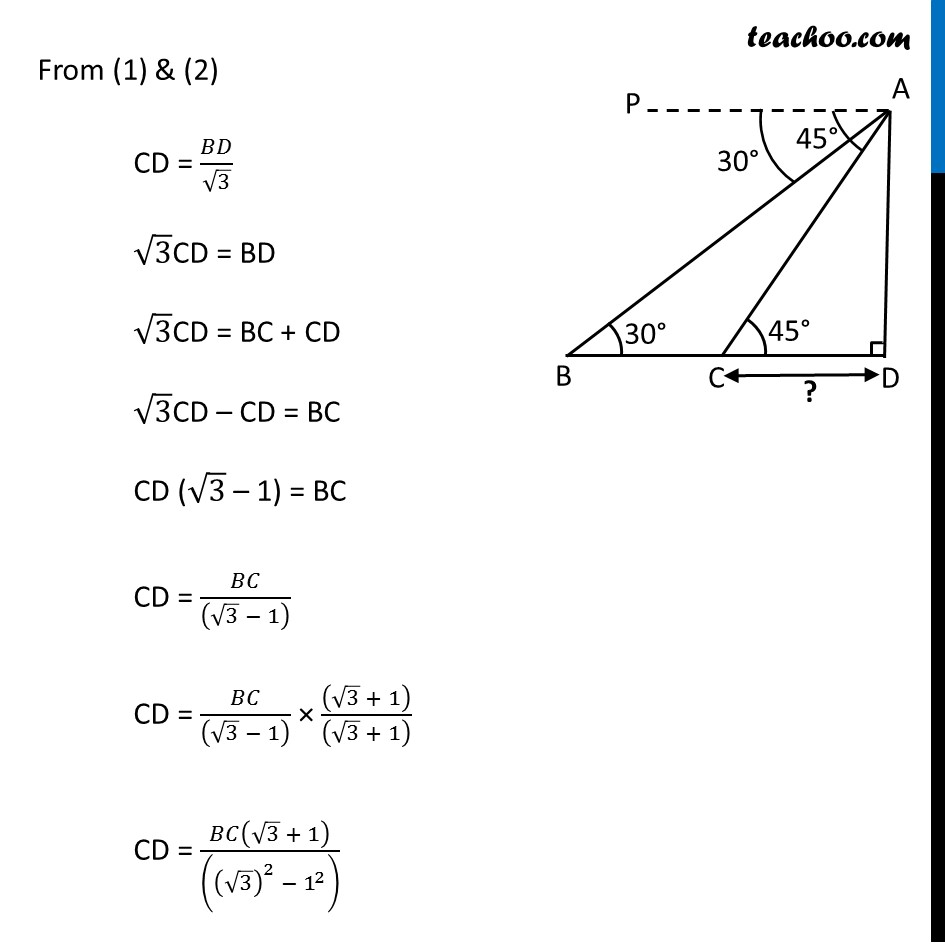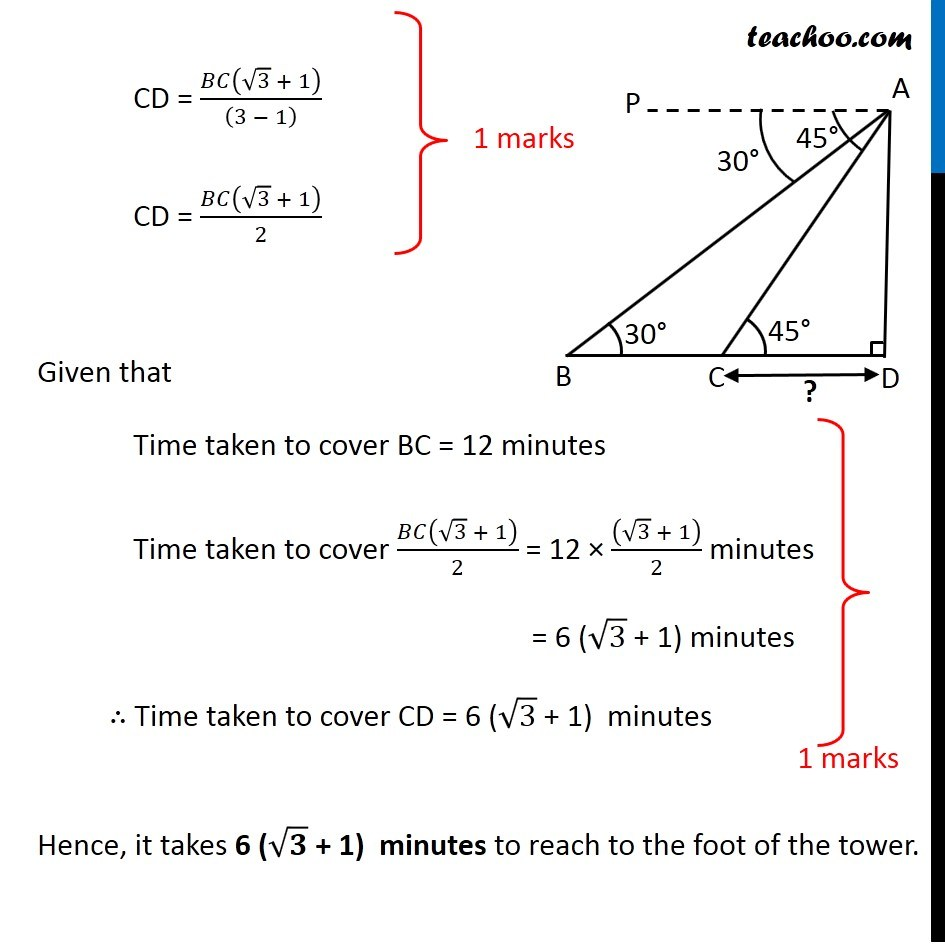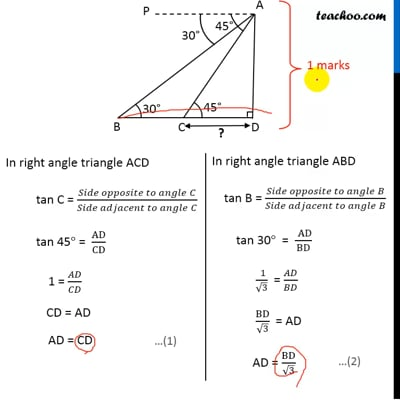This video is only available for Teachoo black users

### Transcript

Question 27 (OR 1st question) A man on the top of a vertical observation tower observes a car moving at a uniform speed coming directly towards it. If it takes 12 minutes for the angle of depression to change from 30° to 45°, how long will the car take to reach the observation tower from this point? Let the man & tower height be AD Given that man sees car first at an angle of depression of 30° So, ∠ PAB = 30° After 12 minutes, the man sees car at an angle of depression of 45° So, ∠ PAC = 45° Now, tower is vertical, So, ∠ ADB = 90° Also, Lines PA & BD are parallel And AB is the transversal ∠ ABD = ∠ PAB (Alternate Angles) So, ∠ ABD = 30° Lines PA & BD are parallel And AC is the transversal ∠ ACD = ∠ PAC (Alternate Angles) So, ∠ ACD = 45° In right angle triangle ACD tan C = (𝑆𝑖𝑑𝑒 𝑜𝑝𝑝𝑜𝑠𝑖𝑡𝑒 𝑡𝑜 𝑎𝑛𝑔𝑙𝑒" " 𝐶)/(𝑆𝑖𝑑𝑒 𝑎𝑑𝑗𝑎𝑐𝑒𝑛𝑡 𝑡𝑜 𝑎𝑛𝑔𝑙𝑒" " 𝐶) tan 45° = AD/CD 1 = 𝐴𝐷/𝐶𝐷 CD = AD AD = CD In right angle triangle ABD tan B = (𝑆𝑖𝑑𝑒 𝑜𝑝𝑝𝑜𝑠𝑖𝑡𝑒 𝑡𝑜 𝑎𝑛𝑔𝑙𝑒" " 𝐵)/(𝑆𝑖𝑑𝑒 𝑎𝑑𝑗𝑎𝑐𝑒𝑛𝑡 𝑡𝑜 𝑎𝑛𝑔𝑙𝑒" " 𝐵) tan 30° = (" " AD)/BD (" " 1)/√3 = 𝐴𝐷/𝐵𝐷 BD/√3 = AD AD = BD/√3 From (1) & (2) CD = 𝐵𝐷/√3 √3CD = BD √3CD = BC + CD √3CD – CD = BC CD (√3 – 1) = BC CD = 𝐵𝐶/((√3 − 1) ) CD = 𝐵𝐶/((√3 − 1) ) × ((√3 + 1))/((√3 + 1) ) CD = 𝐵𝐶(√3 + 1)/(((√3)^2 − 1^2 ) ) CD = 𝐵𝐶(√3 + 1)/((3 − 1) ) CD = 𝐵𝐶(√3 + 1)/2 Given that Time taken to cover BC = 12 minutes Time taken to cover 𝐵𝐶(√3 + 1)/2 = 12 × ((√3 + 1))/2 minutes = 6 (√3 + 1) minutes ∴ Time taken to cover CD = 6 (√3 + 1) minutes Hence, it takes 6 (√𝟑 + 1) minutes to reach to the foot of the tower.